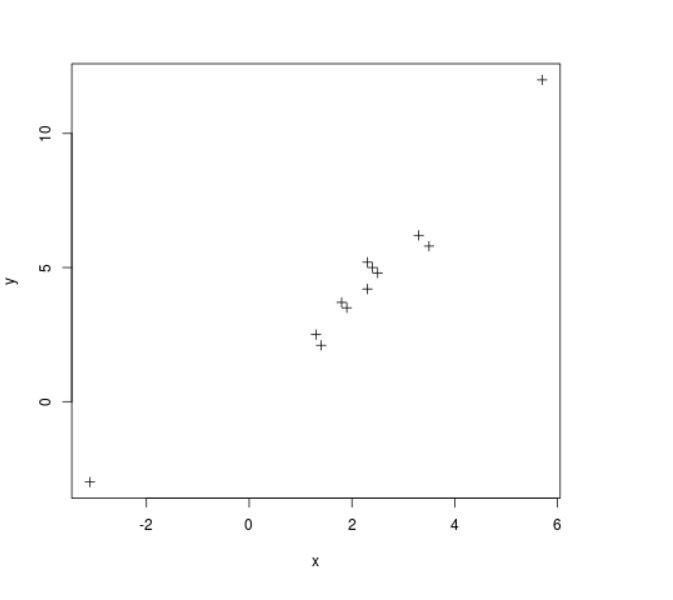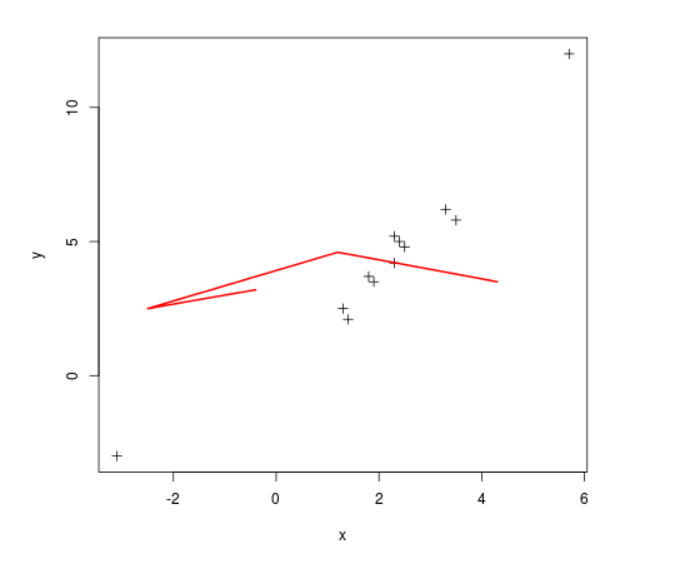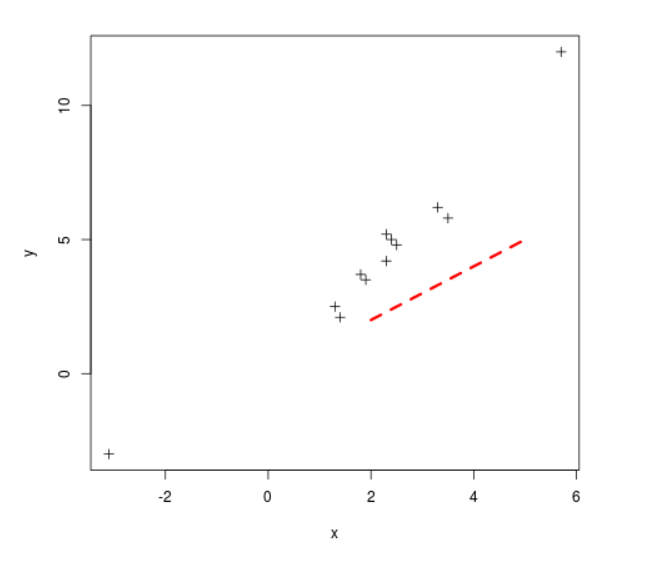# Addition of Lines to a Plot in R Programming – lines() Function

`lines()` function in R Language is used to add lines of different types, color and width to an existing plot.

Syntax: lines(x, y, col, lwd, lty)

Parameters:
x, y: Vector of coordinates
col: Color of line
lwd: Width of line
lty: Type of line

Sample Scatter plot:

 `# R program to create a scatter plot ` `  `  `# Creating coordinate vectors ` `x <``-` `c(``1.3``, ``3.5``, ``1.4``, ``-``3.1``, ``5.7``, ``2.4``, ``3.3``, ``2.5``, ``2.3``, ``1.9``, ``1.8``, ``2.3``) ` `y <``-` `c(``2.5``, ``5.8``, ``2.1``, ``-``3``, ``12``, ``5``, ``6.2``, ``4.8``, ``4.2``, ``3.5``, ``3.7``, ``5.2``) ` `  `  `# Plotting the graph ` `plot(x, y, cex ``=` `1``, pch ``=` `3``, xlab ``=``"x"``, ylab ``=``"y"``, col ``=``"black"``) `Example 1:

 `# R program to add lines into plots ` `  `  `# Creating coordinate vectors ` `x <``-` `c(``1.3``, ``3.5``, ``1.4``, ``-``3.1``, ``5.7``, ``2.4``, ``3.3``, ``2.5``, ``2.3``, ``1.9``, ``1.8``, ``2.3``) ` `y <``-` `c(``2.5``, ``5.8``, ``2.1``, ``-``3``, ``12``, ``5``, ``6.2``, ``4.8``, ``4.2``, ``3.5``, ``3.7``, ``5.2``) ` `  `  `# Plotting the graph ` `plot(x, y, cex ``=` `1``, pch ``=` `3``, xlab ``=``"x"``, ylab ``=``"y"``, col ``=``"black"``) ` ` `  `# Creating coordinate vectors ` `x2 <``-` `c(``4.3``, ``1.2``, ``-``2.5``, ``-``0.4``) ` `y2 <``-` `c(``3.5``, ``4.6``, ``2.5``, ``3.2``) ` ` `  `# Plotting a line ` `lines(x2, y2, col ``=` `"red"``, lwd ``=` `2``, lty ``=` `1``) `

Output:Example 2:

 `# R program to add lines into plots ` `  `  `# Creating coordinate vectors ` `x <``-` `c(``1.3``, ``3.5``, ``1.4``, ``-``3.1``, ``5.7``, ``2.4``, ``3.3``, ``2.5``, ``2.3``, ``1.9``, ``1.8``, ``2.3``) ` `y <``-` `c(``2.5``, ``5.8``, ``2.1``, ``-``3``, ``12``, ``5``, ``6.2``, ``4.8``, ``4.2``, ``3.5``, ``3.7``, ``5.2``) ` `  `  `# Plotting the graph ` `plot(x, y, cex ``=` `1``, pch ``=` `3``, xlab ``=``"x"``, ylab ``=``"y"``, col ``=``"black"``) ` ` `  `# Creating coordinate vectors ` `x2 <``-` `c(``2``, ``3``, ``4``, ``5``) ` `y2 <``-` `c(``2``, ``3``, ``4``, ``5``) ` ` `  `# Plotting a line ` `lines(x2, y2, col ``=` `"red"``, lwd ``=` `3``, lty ``=` `2``) `

Output:My Personal Notes arrow_drop_upCheck out this Author's contributed articles.

If you like GeeksforGeeks and would like to contribute, you can also write an article using contribute.geeksforgeeks.org or mail your article to contribute@geeksforgeeks.org. See your article appearing on the GeeksforGeeks main page and help other Geeks.

Please Improve this article if you find anything incorrect by clicking on the "Improve Article" button below.

Article Tags :

Be the First to upvote.

Please write to us at contribute@geeksforgeeks.org to report any issue with the above content.